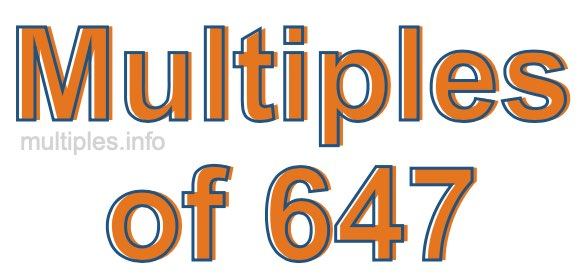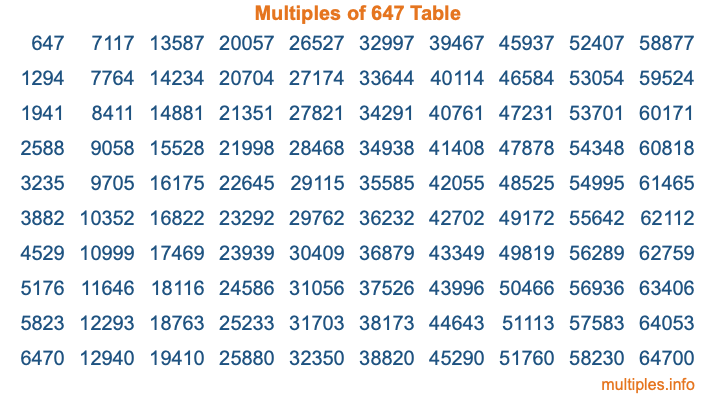Multiples of 647Welcome to the Multiples of 647 page. Here we will first teach you everything you will ever need to know about the multiples of 647, and then give you a study guide summary of everything we taught you to make sure you remember it all. Use this page to look up facts and learn information about the multiples of 647. This page will make you a multiples of six hundred forty-seven expert!

Definition of Multiples of 647
Multiples of 647 are all the numbers that when divided by 647 equal an integer. Each of the multiples of 647 are called a multiple. A multiple of 647 is created by multiplying 647 by an integer.

Therefore, to create a list of multiples of 647, you start with 1 multiplied by 647, then 2 multiplied by 647, then 3 multiplied by 647, and so on for as long as you want. Thus, the list of the first five multiples of 647 is 647, 1294, 1941, 2588, and 3235. To see a larger list of multiples of 647, see the printable image of Multiples of 647 further down on this page. We also have a category where you can choose any nth multiple of 647.

Multiples of 647 Checker
The Multiples of 647 Checker below checks to see if any number of your choice is a multiple of 647. In other words, it checks to see if there is any number (integer) that when multiplied by 647 will equal your number. To do that, we divide your number by 647. If the the quotient is an integer, then your number is a multiple of 647.

Is  a multiple of 647?

Least Common Multiple of 647 and ...
A Least Common Multiple (LCM) is the lowest multiple that two or more numbers have in common. This is also called the smallest common multiple or lowest common multiple and is useful to know when you are adding our subtracting fractions. Enter one or more numbers below (647 is already entered) to find the LCM.

Check out our LCM Calculator if you need more details about the Least Common Multiple or if you need the LCM for different numbers for adding and subtraction fractions.

nth Multiple of 647
As we stated above, 647 is the first multiple of 647, 1294 is the second multiple of 647, 1941 is the third multiple of 647, and so on. Enter a number below to find the nth multiple of 647.

th multiple of 647

Multiples of 647 vs Factors of 647
647 is a multiple of 647 and a factor of 647, but that is where the similarities end. All postive multiples of 647 are 647 or greater than 647. All positive factors of 647 are 647 or less than 647.

Below is the beginning list of multiples of 647 and the factors of 647 so you can compare:

Multiples of 647: 647, 1294, 1941, 2588, 3235, etc.

Factors of 647: 1, 647

As you can see, the multiples of 647 are all the numbers that you can divide by 647 to get a whole number. The factors of 647, on the other hand, are all the whole numbers that you can multiply by another whole number to get 647.

It's also interesting to note that if a number (x) is a factor of 647, then 647 will also be a multiple of that number (x).

Multiples of 647 vs Divisors of 647
The divisors of 647 are all the integers that 647 can be divided by evenly. Below is a list of the divisors of 647.

Divisors of 647: 1, 647

The interesting thing to note here is that if you take any multiple of 647 and divide it by a divisor of 647, you will see that the quotient is an integer.

Multiples of 647 Table
Below is an image of the first 100 multiples of 647 in a table. The table is in chronological order, column by column. The first column has the first ten multiples of 647, the second column has the next ten multiples of 647, and so on.The Multiples of 647 Table is also referred to as the 647 Times Table or Times Table of 647. You are welcome to print out our table for your studies.

Negative Multiples of 647
Although not often discussed or needed in math, it is worth mentioning that you can make a list of negative multiples of 647 by multiplying 647 by -1, then by -2, then by -3, and so on, to get the following list of negative multiples of 647:

-647, -1294, -1941, -2588, -3235, etc.

Multiples of 647 Summary
Below is a summary of important Multiples of 647 facts that we have discussed on this page. To retain the knowledge on this page, we recommend that you read through the summary and explain to yourself or a study partner why they hold true.

There are an infinite number of multiples of 647.

A multiple of 647 divided by 647 will equal a whole number.

647 divided by a factor of 647 equals a divisor of 647.

The nth multiple of 647 is n times 647.

The largest factor of 647 is equal to the first positive multiple of 647.

647 is a multiple of every factor of 647.

647 is a multiple of 647.

A multiple of 647 divided by a divisor of 647 equals an integer.

647 divided by a divisor of 647 equals a factor of 647.

Any integer times 647 will equal a multiple of 647.

Multiples of a Number
Here you can get the multiples of another number, all with the same attention to detail as we did for multiples of 647 on this page.

Multiples of
Multiples of 648
Did you find our page about multiples of six hundred forty-seven educational? Do you want more knowledge? Check out the multiples of the next number on our list!